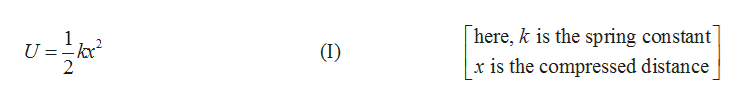# A 3.2 kg block is hanging stationary from the end of a vertical spring that is attached to the ceiling. The elastic potential energy of this spring/mass sytem is 1.5 J. What is the elastic potential energy of the system when the 3.2 kg block is replaced by a 5.7 kg block?

Question
73 views

A 3.2 kg block is hanging stationary from the end of a vertical spring that is attached to the ceiling. The elastic potential energy of this spring/mass sytem is 1.5 J. What is the elastic potential energy of the system when the 3.2 kg block is replaced by a 5.7 kg block?

check_circle

Step 1

The mass of the first block =m1=3.2kg.

The elastic potential energy corresponding to block with mass m1=U1=1.5J.

Let m2 be the second mass, and corresponding potential energy be U2.

Write the expression for the potential energy of the springhelp_outlineImage Transcriptionclosehere, k is the spring constant 1 U =-kx 2 (I) x is the compressed distance fullscreen
Step 2

Write the expression for the force acting on spring.

Step 3

Substitute equation (II) in (I) and write the expression for th...

### Want to see the full answer?

See Solution

#### Want to see this answer and more?

Solutions are written by subject experts who are available 24/7. Questions are typically answered within 1 hour.*

See Solution
*Response times may vary by subject and question.
Tagged in

### Kinematics